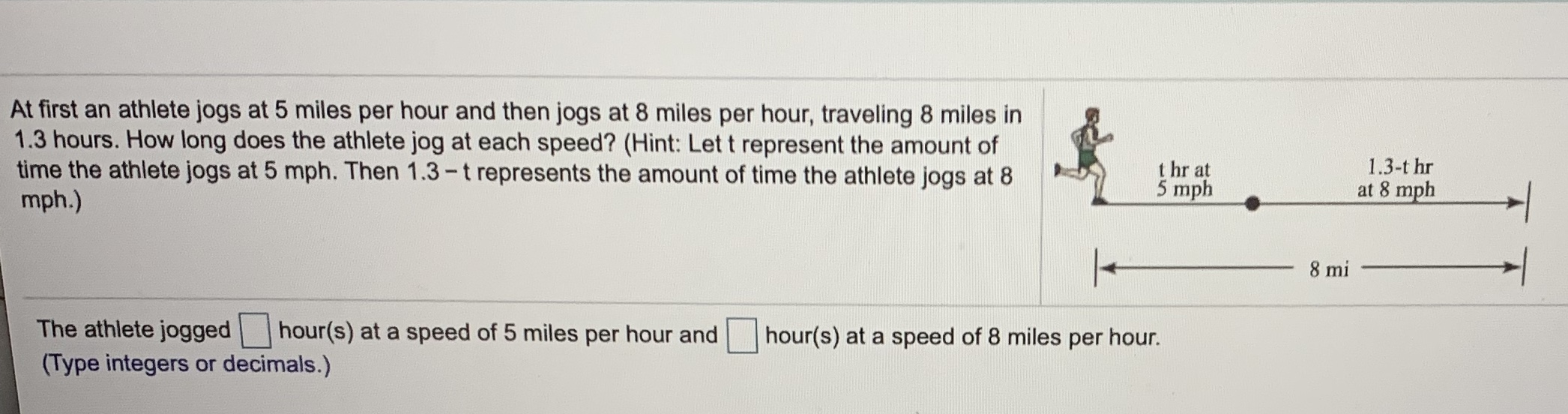# At first an athlete jogs at 5 miles per hour and then jogs at 8 miles per hour, traveling 8 miles in 1.3 hours. How long does the athlete jog at each speed? (Hint: Let t represent the amount of time the athlete jogs at 5 mph. Then 1.3-t represents the amount of time the athlete jogs at 8 mph.) 1.3-t hr t hr at 5 mph at 8 mph 8 mi The athlete jogged hour(s) at a speed of 5 miles per hour and hour(s) at a speed of 8 miles per hour. (Type integers or decimals.)

Questionhelp_outlineImage TranscriptioncloseAt first an athlete jogs at 5 miles per hour and then jogs at 8 miles per hour, traveling 8 miles in 1.3 hours. How long does the athlete jog at each speed? (Hint: Let t represent the amount of time the athlete jogs at 5 mph. Then 1.3-t represents the amount of time the athlete jogs at 8 mph.) 1.3-t hr t hr at 5 mph at 8 mph 8 mi The athlete jogged hour(s) at a speed of 5 miles per hour and hour(s) at a speed of 8 miles per hour. (Type integers or decimals.) fullscreen

### Want to see this answer and more?

Experts are waiting 24/7 to provide step-by-step solutions in as fast as 30 minutes!*

*Response times may vary by subject and question complexity. Median response time is 34 minutes for paid subscribers and may be longer for promotional offers.
Tagged in
Math
Algebra

### Other